# Filters

Provides a demonstration of using different IIR, FIR and Kernel-based Filters available in JDSP

## Butterworth Filter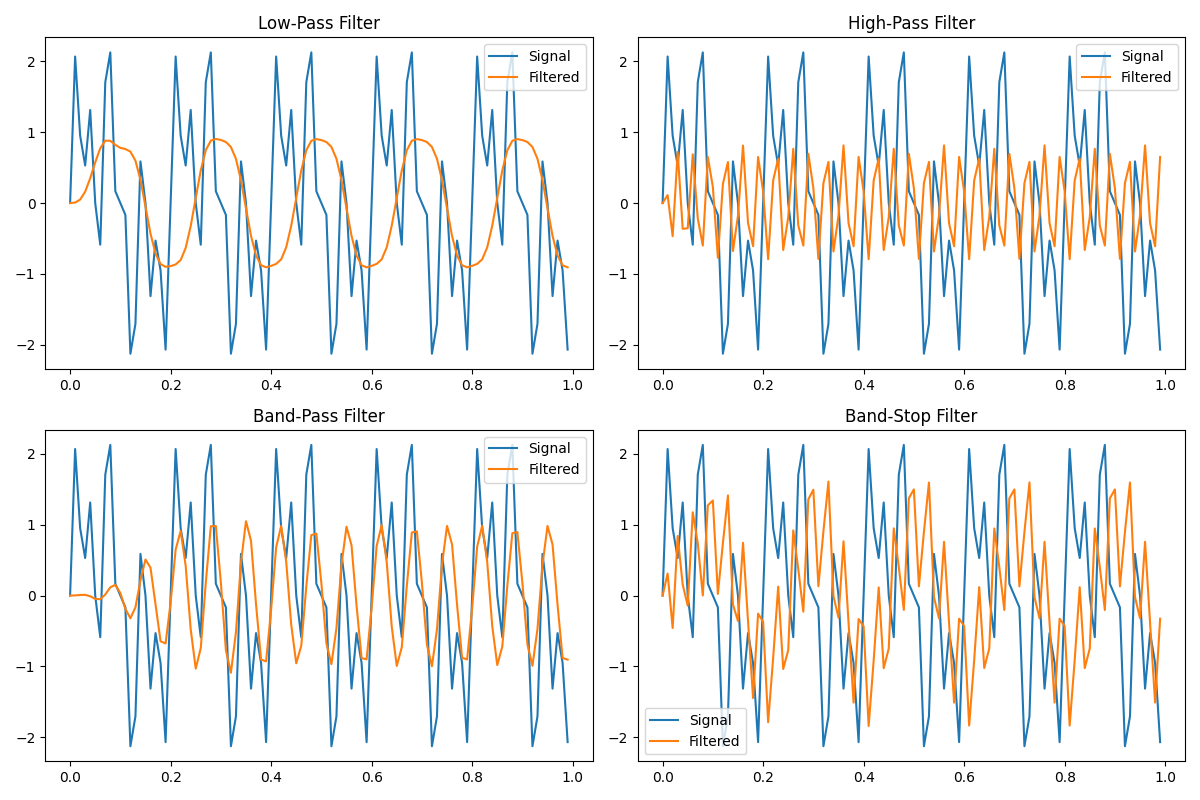#### High Pass Filter

```                    ```
int Fs = 100; //Sampling Frequency in Hz
int order = 4; //order of the filter
int cutOff = 9; //Cut-off Frequency
Butterworth flt = new Butterworth(Fs);
double[] result = flt.lowPassFilter(signal, order, cutOff); //get the result after filtering
```
```
```                    ```
int Fs = 100; //Sampling Frequency in Hz
int order = 4; //order of the filter
int cutOff = 29; //Cut-off Frequency
Butterworth flt = new Butterworth(Fs);
double[] result = flt.highPassFilter(signal, order, cutOff); //get the result after filtering
```
```

#### Band Stop Filter

```                    ```
int Fs = 100; //Sampling Frequency in Hz
int order = 4; //order of the filter
int lowCutOff = 12; //Lower Cut-off Frequency
int highCutOff = 18; //Higher Cut-off Frequency
Butterworth flt = new Butterworth(Fs);
double[] result = flt.bandPassFilter(signal, order, lowCutOff, highCutOff); //get the result after filtering
```
```
```                    ```
int Fs = 100; //Sampling Frequency in Hz
int order = 4; //order of the filter
int lowCutOff = 7; //Lower Cut-off Frequency
int highCutOff = 28; //Higher Cut-off Frequency
Butterworth flt = new Butterworth(Fs);
double[] result = flt.bandStopFilter(signal, order, lowCutOff, highCutOff); //get the result after filtering
```
```

## Chebyshev Type I Filter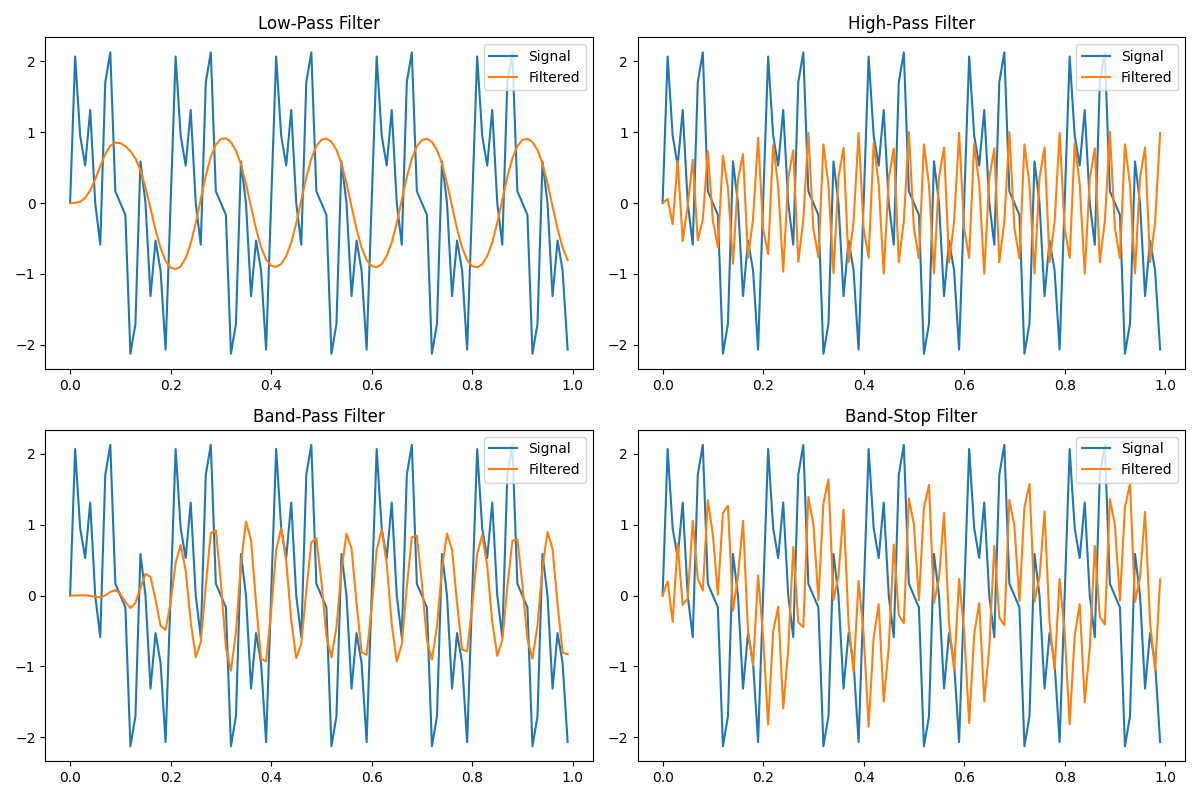#### High Pass Filter

```                    ```
int filterType = 1; //Can be 1 (for type 1) or 2 (for type 2)
double rippleFactor = 1; //maximum ripple allowed below unity gain
int Fs = 100; //Sampling Frequency in Hz
int order = 4; //order of the filter
int cutOff = 9; //Cut-off Frequency
Chebyshev flt = new Chebyshev(Fs, rippleFactor, filterType);
double[] result = flt.lowPassFilter(signal, order, cutOff); //get the result after filtering
```
```
```                    ```
int filterType = 1; //Can be 1 (for type 1) or 2 (for type 2)
double rippleFactor = 1; //maximum ripple allowed below unity gain
int Fs = 100; //Sampling Frequency in Hz
int order = 4; //order of the filter
int cutOff = 29; //Cut-off Frequency
Chebyshev flt = new Chebyshev(Fs, rippleFactor, filterType);
double[] result = flt.highPassFilter(signal, order, cutOff); //get the result after filtering
```
```

#### Band Stop Filter

```                    ```
int filterType = 1; //Can be 1 (for type 1) or 2 (for type 2)
double rippleFactor = 1; //maximum ripple allowed below unity gain
int Fs = 100; //Sampling Frequency in Hz
int order = 4; //order of the filter
int lowCutOff = 12; //Lower Cut-off Frequency
int highCutOff = 18; //Higher Cut-off Frequency
Chebyshev flt = new Chebyshev(Fs, rippleFactor, filterType);
double[] result = flt.BandPassFilter(signal, order, lowCutOff, highCutOff); //get the result after filtering
```
```
```                    ```
int filterType = 1; //Can be 1 (for type 1) or 2 (for type 2)
double rippleFactor = 1; //maximum ripple allowed below unity gain
int Fs = 100; //Sampling Frequency in Hz
int order = 4; //order of the filter
int lowCutOff = 7; //Lower Cut-off Frequency
int highCutOff = 28; //Higher Cut-off Frequency
Chebyshev flt = new Chebyshev(Fs, rippleFactor, filterType);
double[] result = flt.bandStopFilter(signal, order, lowCutOff, highCutOff); //get the result after filtering
```
```

## Chebyshev Type II Filter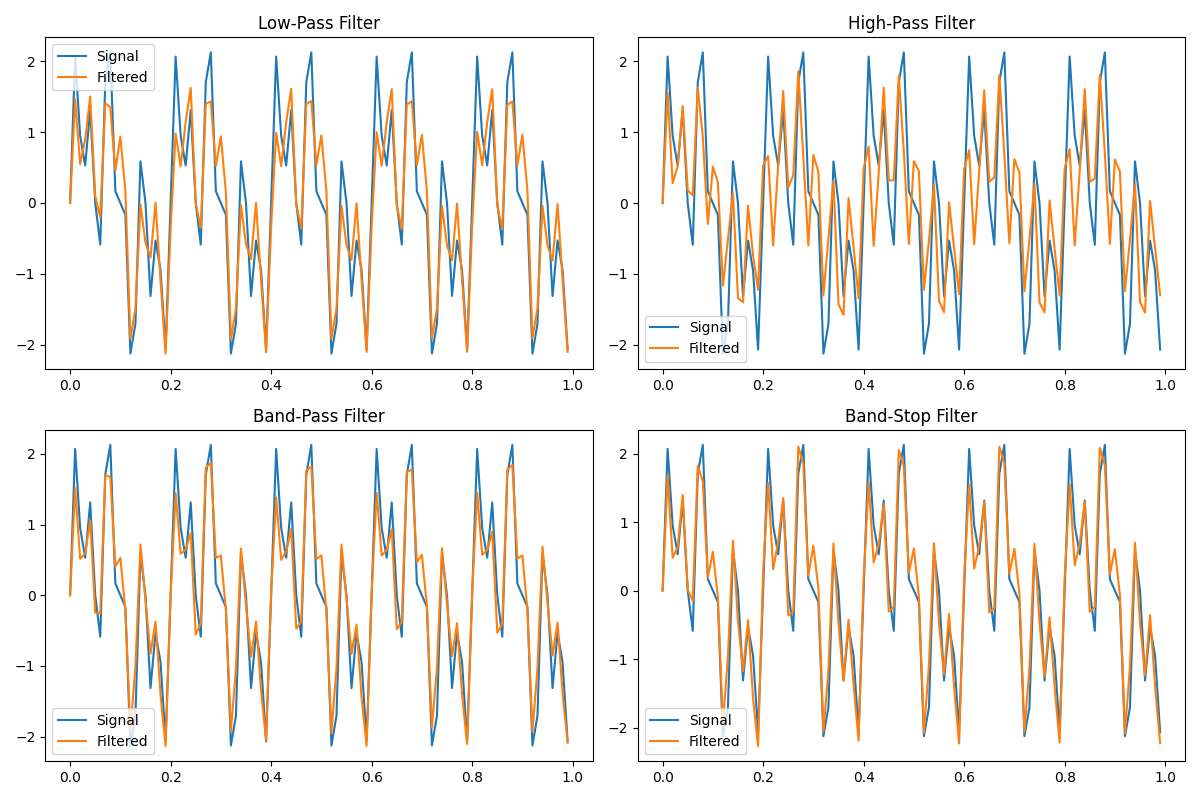#### High Pass Filter

```                    ```
int filterType = 2; //Can be 1 (for type 1) or 2 (for type 2)
double rippleFactor = 1; //maximum ripple allowed below unity gain
int Fs = 100; //Sampling Frequency in Hz
int order = 4; //order of the filter
int cutOff = 9; //Cut-off Frequency
Chebyshev flt = new Chebyshev(Fs, rippleFactor, filterType);
double[] result = flt.lowPassFilter(signal, order, cutOff); //get the result after filtering
```
```
```                    ```
int filterType = 2; //Can be 1 (for type 1) or 2 (for type 2)
double rippleFactor = 1; //maximum ripple allowed below unity gain
int Fs = 100; //Sampling Frequency in Hz
int order = 4; //order of the filter
int cutOff = 29; //Cut-off Frequency
Chebyshev flt = new Chebyshev(Fs, rippleFactor, filterType);
double[] result = flt.highPassFilter(signal, order, cutOff); //get the result after filtering
```
```

#### Band Stop Filter

```                    ```
int filterType = 2; //Can be 1 (for type 1) or 2 (for type 2)
double rippleFactor = 1; //maximum ripple allowed below unity gain
int Fs = 100; //Sampling Frequency in Hz
int order = 4; //order of the filter
int lowCutOff = 12; //Lower Cut-off Frequency
int highCutOff = 18; //Higher Cut-off Frequency
Chebyshev flt = new Chebyshev(Fs, rippleFactor, filterType);
double[] result = flt.bandPassFilter(signal, order, lowCutOff, highCutOff); //get the result after filtering
```
```
```                    ```
int filterType = 2; //Can be 1 (for type 1) or 2 (for type 2)
double rippleFactor = 1; //maximum ripple allowed below unity gain
int Fs = 100; //Sampling Frequency in Hz
int order = 4; //order of the filter
int lowCutOff = 7; //Lower Cut-off Frequency
int highCutOff = 28; //Higher Cut-off Frequency
Chebyshev flt = new Chebyshev(Fs, rippleFactor, filterType);
double[] result = flt.bandStopFilter(signal, order, lowCutOff, highCutOff); //get the result after filtering
```
```

## Bessel Filter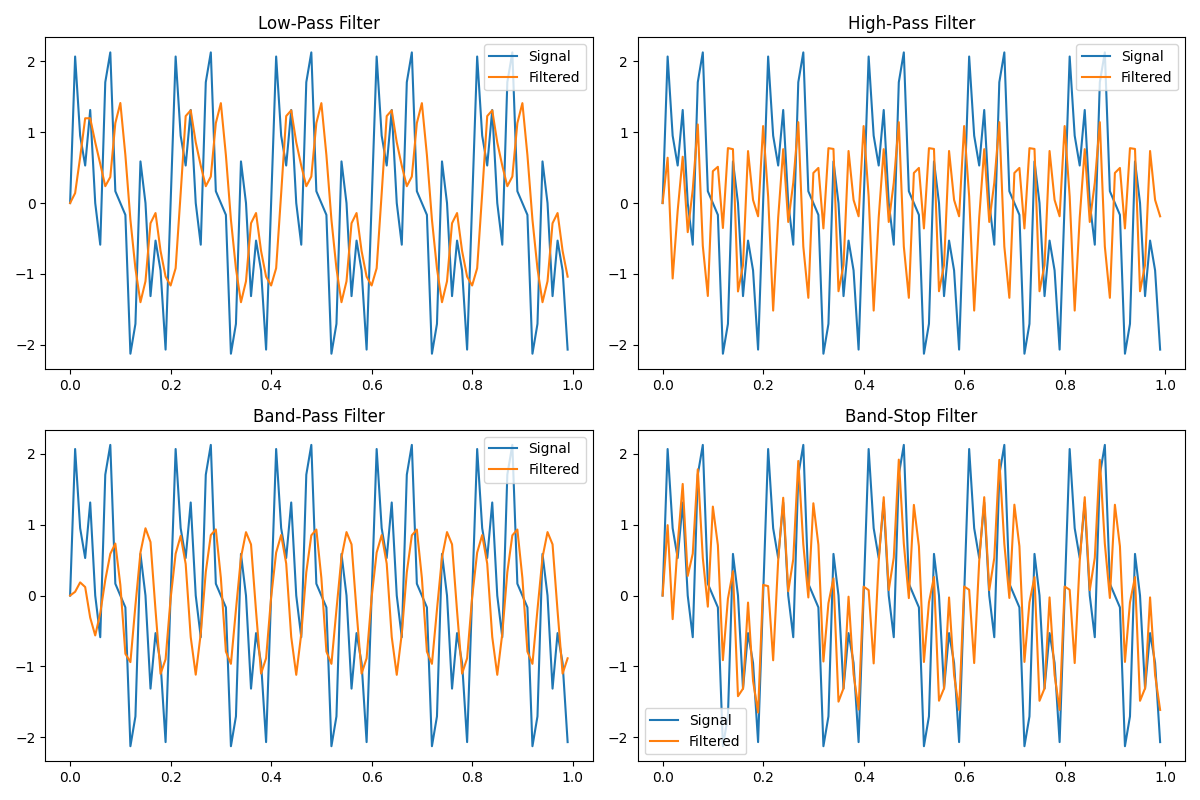#### High Pass Filter

```                    ```
int Fs = 100; //Sampling Frequency in Hz
int order = 4; //order of the filter
int cutOff = 9; //Cut-off Frequency
Bessel flt = new Bessel(Fs);
double[] result = flt.lowPassFilter(signal, order, cutOff); //get the result after filtering
```
```
```                    ```
int Fs = 100; //Sampling Frequency in Hz
int order = 4; //order of the filter
int cutOff = 29; //Cut-off Frequency
Bessel flt = new Bessel(Fs);
double[] result = flt.highPassFilter(signal, order, cutOff); //get the result after filtering
```
```

#### Band Stop Filter

```                    ```
int Fs = 100; //Sampling Frequency in Hz
int order = 4; //order of the filter
int lowCutOff = 12; //Lower Cut-off Frequency
int highCutOff = 18; //Higher Cut-off Frequency
Bessel flt = new Bessel(Fs);
double[] result = flt.bandPassFilter(signal, order, lowCutOff, highCutOff); //get the result after filtering
```
```
```                    ```
int Fs = 100; //Sampling Frequency in Hz
int order = 4; //order of the filter
int lowCutOff = 7; //Lower Cut-off Frequency
int highCutOff = 28; //Higher Cut-off Frequency
Bessel flt = new Bessel(signal, Fs); //signal is of type double[]
double[] result = flt.bandStopFilter(order, lowCutOff, highCutOff); //get the result after filtering
```
```

## Windowed FIR Filter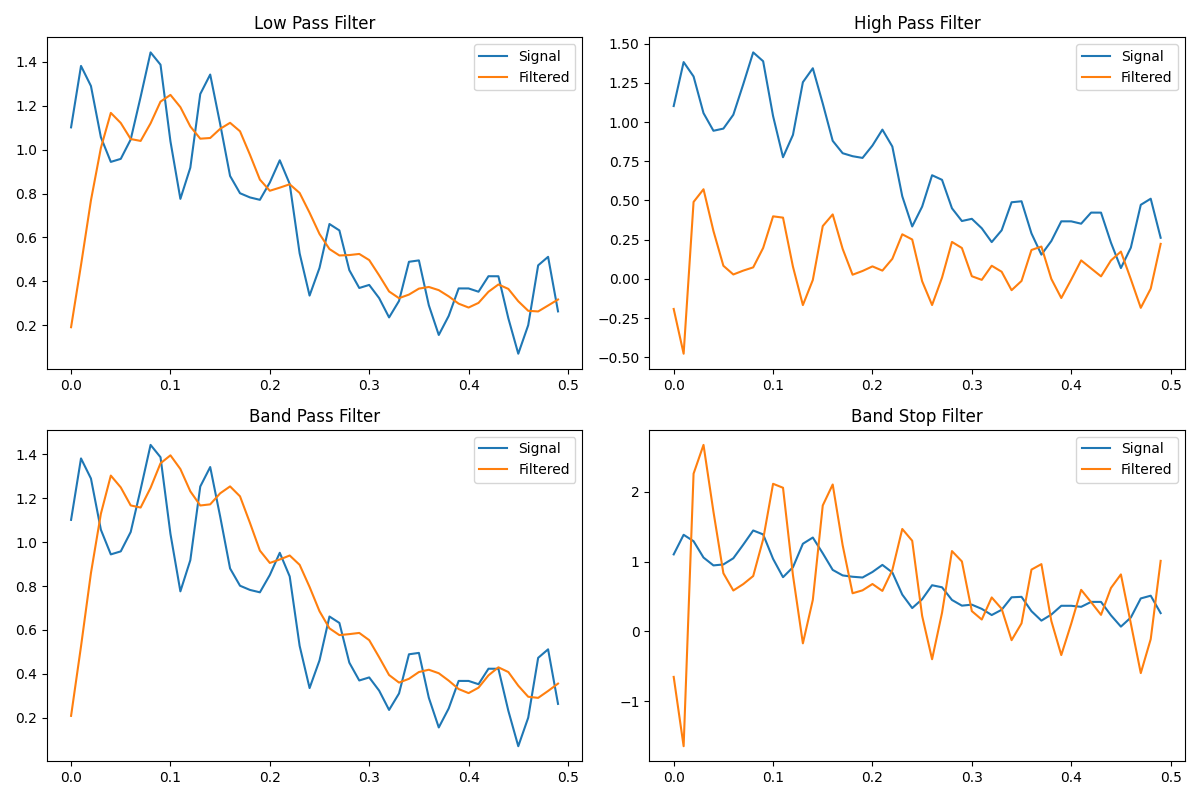#### High Pass Filter

```                    ```
double width = 5;
double samplingRate = 100;
int taps = 5;
double[] cutoff = {10.0};

FIRWin1 fw = new FIRWin1(taps, width, samplingRate);
double[] outCoeffs = fw.computeCoefficients(cutoff, FIRWin1.FIRfilterType.LOWPASS, true);
double[] filteredX = fw.firfilter(outCoeffs, signal);
```
```
```                    ```
double width = 5;
double samplingRate = 100;
int taps = 5;
double[] cutoff = {10.0};

FIRWin1 fw = new FIRWin1(taps, width, samplingRate);
double[] outCoeffs = fw.computeCoefficients(cutoff, FIRWin1.FIRfilterType.HIGHPASS, true);
double[] filteredX = fw.firfilter(outCoeffs, signal);
```
```

#### Band Stop Filter

```                    ```
double width = 5;
double samplingRate = 100;
int taps = 5;
double[] cutoff = {1.0, 10.0};

FIRWin1 fw = new FIRWin1(taps, width, samplingRate);
double[] outCoeffs = fw.computeCoefficients(cutoff, FIRWin1.FIRfilterType.BANDPASS, true);
double[] filteredX = fw.firfilter(outCoeffs, signal);
```
```
```                    ```
double width = 2;
double samplingRate = 100;
int taps = 5;
double[] cutoff = {1.0, 10.0};

FIRWin1 fw = new FIRWin1(taps, width, samplingRate);
double[] outCoeffs = fw.computeCoefficients(cutoff, FIRWin1.FIRfilterType.BANDSTOP, true);
double[] filteredX = fw.firfilter(outCoeffs, signal);
```
```

## Windowed FIR Filter with Gain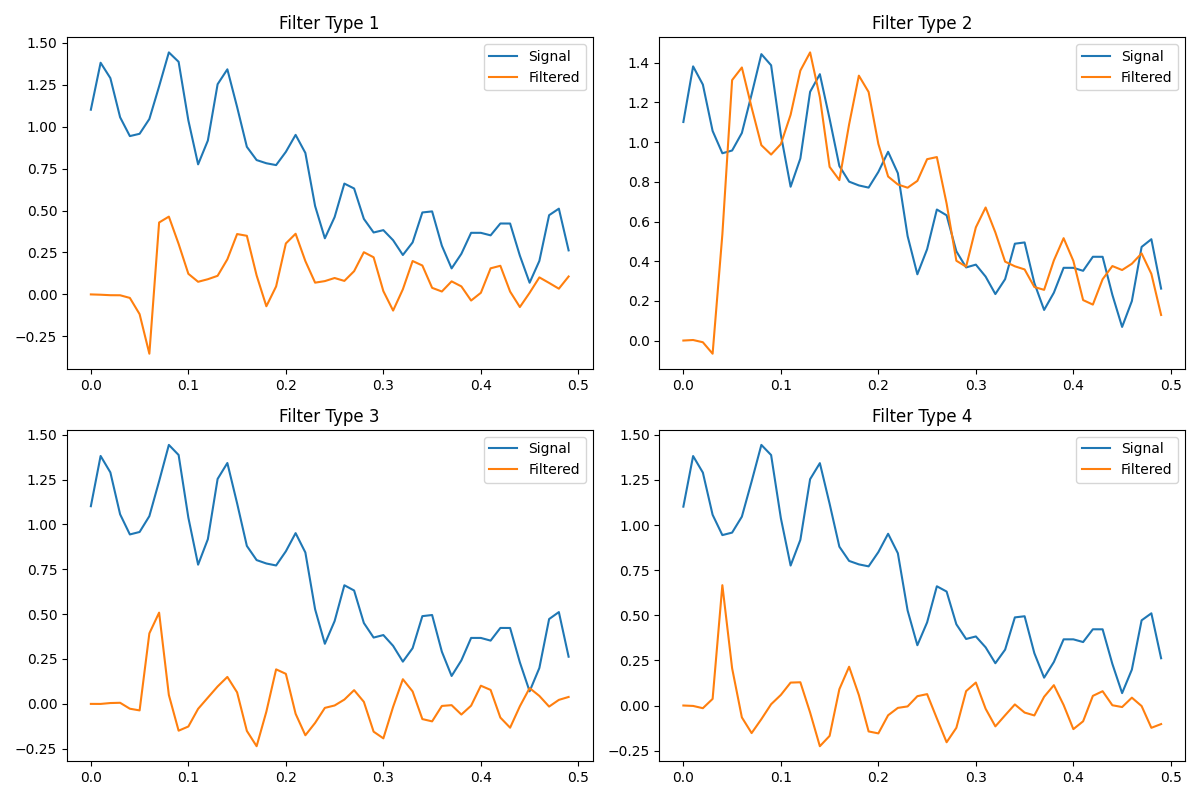#### Type 2 Filter

```                    ```
double samplingRate = 100;
double[] freqs = {0.0, 25, 50};
double[] gains = {0.0, 1.0, 1.0};
int taps = 15;

FIRWin2 fw2 = new FIRWin2(taps, samplingRate);
double[] outCoeffs = fw2.computeCoefficients(freqs, gains);
double[] filteredX = fw2.firfilter(outCoeffs, signal);
```
```
```                    ```
double samplingRate = 100;
double[] freqs = {0.0, 25, 50};
double[] gains = {1.0, 1.0, 0.0};
int taps = 10;

FIRWin2 fw2 = new FIRWin2(taps, samplingRate);
double[] outCoeffs = fw2.computeCoefficients(freqs, gains);
double[] filteredX = fw2.firfilter(outCoeffs, signal);
```
```

#### Type 4 Filter

```                    ```
double samplingRate = 100;
double[] freqs = {0.0, 25, 50};
double[] gains = {0.0, 1.0, 0.0};
int taps = 15;

FIRWin2 fw2 = new FIRWin2(taps, samplingRate, true);
double[] outCoeffs = fw2.computeCoefficients(freqs, gains);
double[] filteredX = fw2.firfilter(outCoeffs, signal);
```
```
```                    ```
double samplingRate = 100;
double[] freqs = {0.0, 25, 50};
double[] gains = {0.0, 1.0, 1.0};
int taps = 10;

FIRWin2 fw2 = new FIRWin2(taps, samplingRate, true);
double[] outCoeffs = fw2.computeCoefficients(freqs, gains);
double[] filteredX = fw2.firfilter(outCoeffs, signal);

```
```

## Least Squares Optimised FIR Filter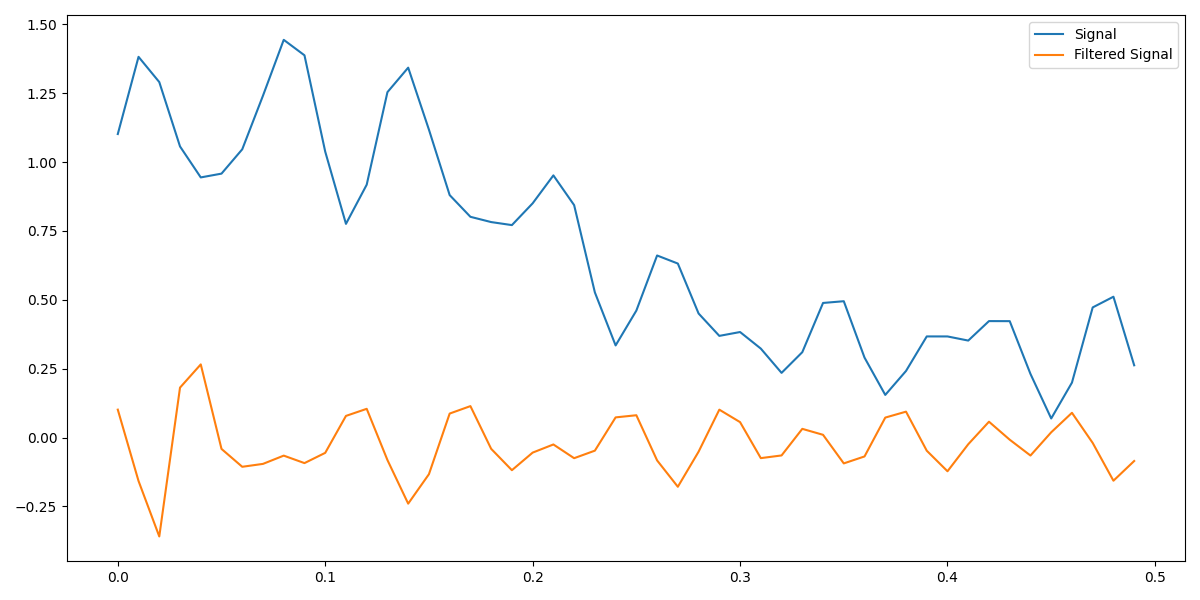```                    ```
double samplingRate = 100;
double[] freqs = {0.0, 1.0, 2.0, 4.0, 4.5, 5.0};
double[] gains = {0.0, 0.0, 1.0, 1.0, 0.0, 0.0};
int taps = 7;

FIRLS fwls = new FIRLS(taps, samplingRate);
double[] coefficients = fwls.computeCoefficients(freqs, gains);
double[] out = fwls.firfilter(coefficients, signal);
```
```

## Median Filter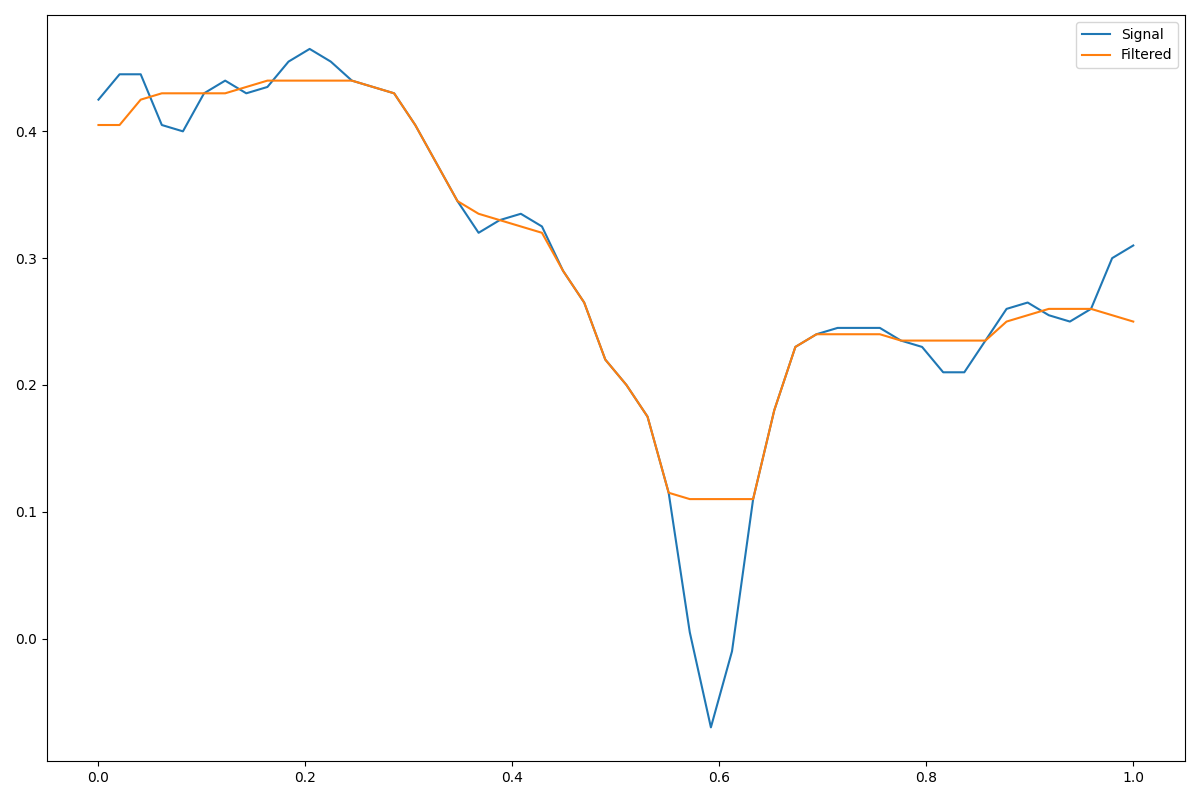```                    ```
int windowSize = 7; //can be anything less than length of signal
MedianFilter mf = new MedianFilter(windowSize);
double[] out = mf.filter(signal); //get the result after filtering
```
```

## Savitzky–Golay Filter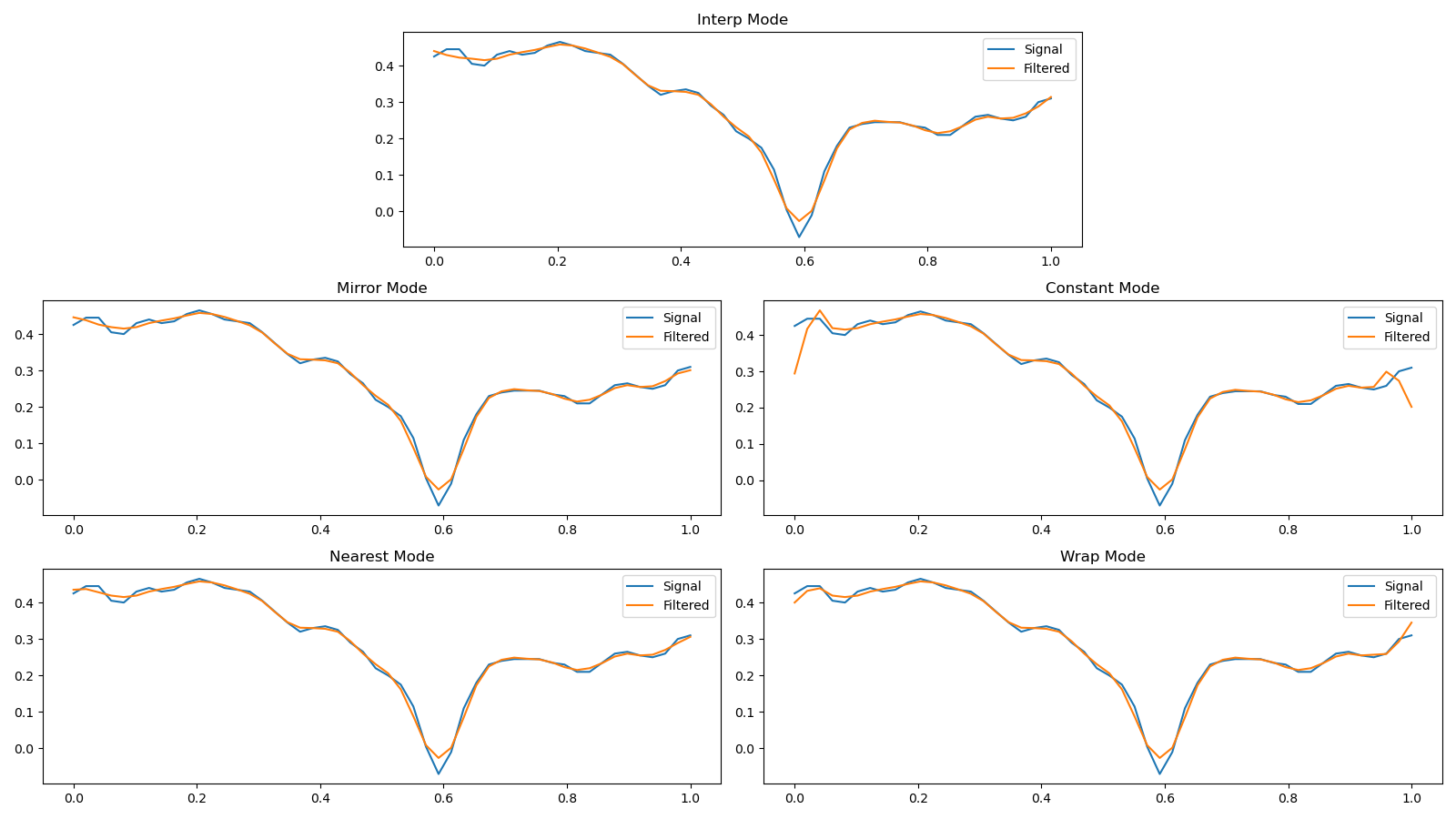#### Constant Mode

```                    ```
String mode = "mirror" //can be any of the operating modes described above
int windowSize = 5; //can be anything less than length of signal
int polyOrder = 2; //Order of the polynomial used to generate coefficients
int deriv = 0; // Order of derivative
int delta = 1; // Spacing of samples to which filter is applied
Savgol s1 = new Savgol(windowSize, polyOrder, deriv, delta);
// ALSO: Savgol s1 = new Savgol(windowSize, polyOrder) set deriv=0, delta=1 by DEFAULT
double[] out = s1.filter(signal, mode); //get the result after filtering
```
```
```                    ```
String mode = "constant" //can be any of the operating modes described above
int windowSize = 5; //can be anything less than length of signal
int polyOrder = 2; //Order of the polynomial used to generate coefficients
int deriv = 0; // Order of derivative
int delta = 1; // Spacing of samples to which filter is applied
Savgol s1 = new Savgol(windowSize, polyOrder, deriv, delta);
// ALSO: Savgol s1 = new Savgol(windowSize, polyOrder) set deriv=0, delta=1 by DEFAULT
double[] out = s1.filter(signal, mode); //get the result after filtering
```
```

#### Wrap Mode

```                    ```
String mode = "nearest" //can be any of the operating modes described above
int windowSize = 5; //can be anything less than length of signal
int polyOrder = 2; //Order of the polynomial used to generate coefficients
int deriv = 0; // Order of derivative
int delta = 1; // Spacing of samples to which filter is applied
Savgol s1 = new Savgol(windowSize, polyOrder, deriv, delta);
// ALSO: Savgol s1 = new Savgol(windowSize, polyOrder) set deriv=0, delta=1 by DEFAULT
double[] out = s1.filter(signal, mode); //get the result after filtering
```
```
```                    ```
String mode = "wrap" //can be any of the operating modes described above
int windowSize = 5; //can be anything less than length of signal
int polyOrder = 2; //Order of the polynomial used to generate coefficients
int deriv = 0; // Order of derivative
int delta = 1; // Spacing of samples to which filter is applied
Savgol s1 = new Savgol(windowSize, polyOrder, deriv, delta);
// ALSO: Savgol s1 = new Savgol(windowSize, polyOrder) set deriv=0, delta=1 by DEFAULT
double[] out = s1.filter(signal, mode); //get the result after filtering
```
```

## Wiener Filter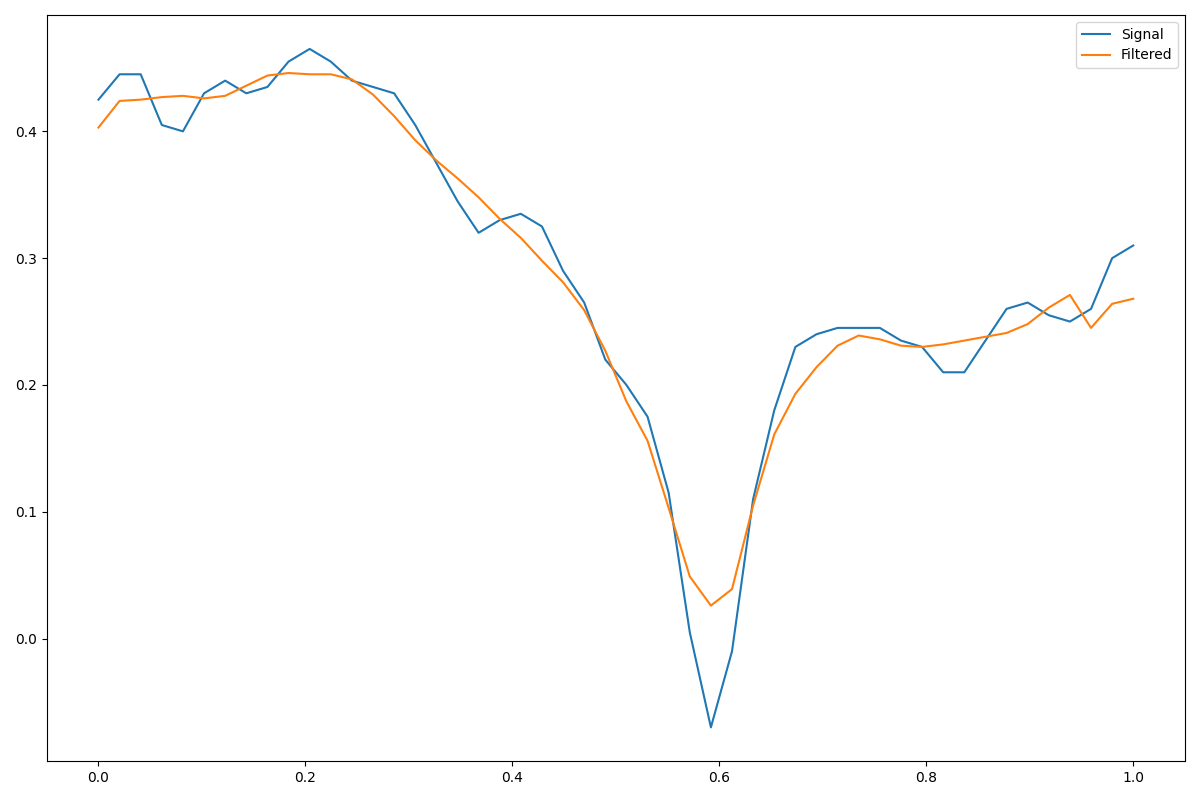```                    ```
int windowSize = 7; //can be anything less than length of signal
Wiener wf = new Wiener(windowSize);
double[] out = wf.filter(signal); //get the result after filtering
```
```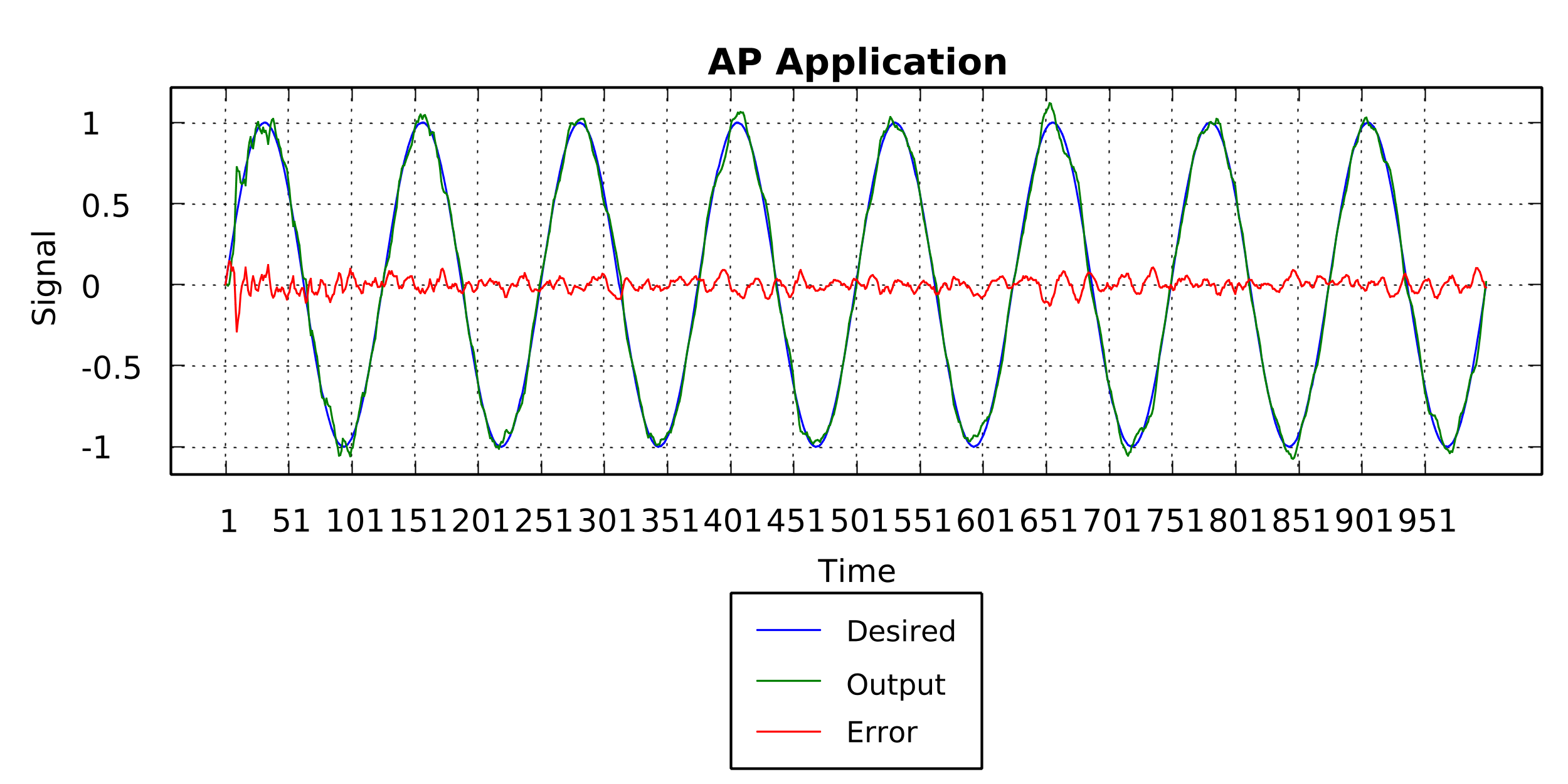```                    ```
int order = 3;
double mu = 0.25;
double eps = 0.001;
int length = 20;

AP filt1 = new AP(length, order, mu, eps, AP.WeightsFillMethod.ZEROS); // Initialising weights to zero
filt1.filter(desired, signal);
```
```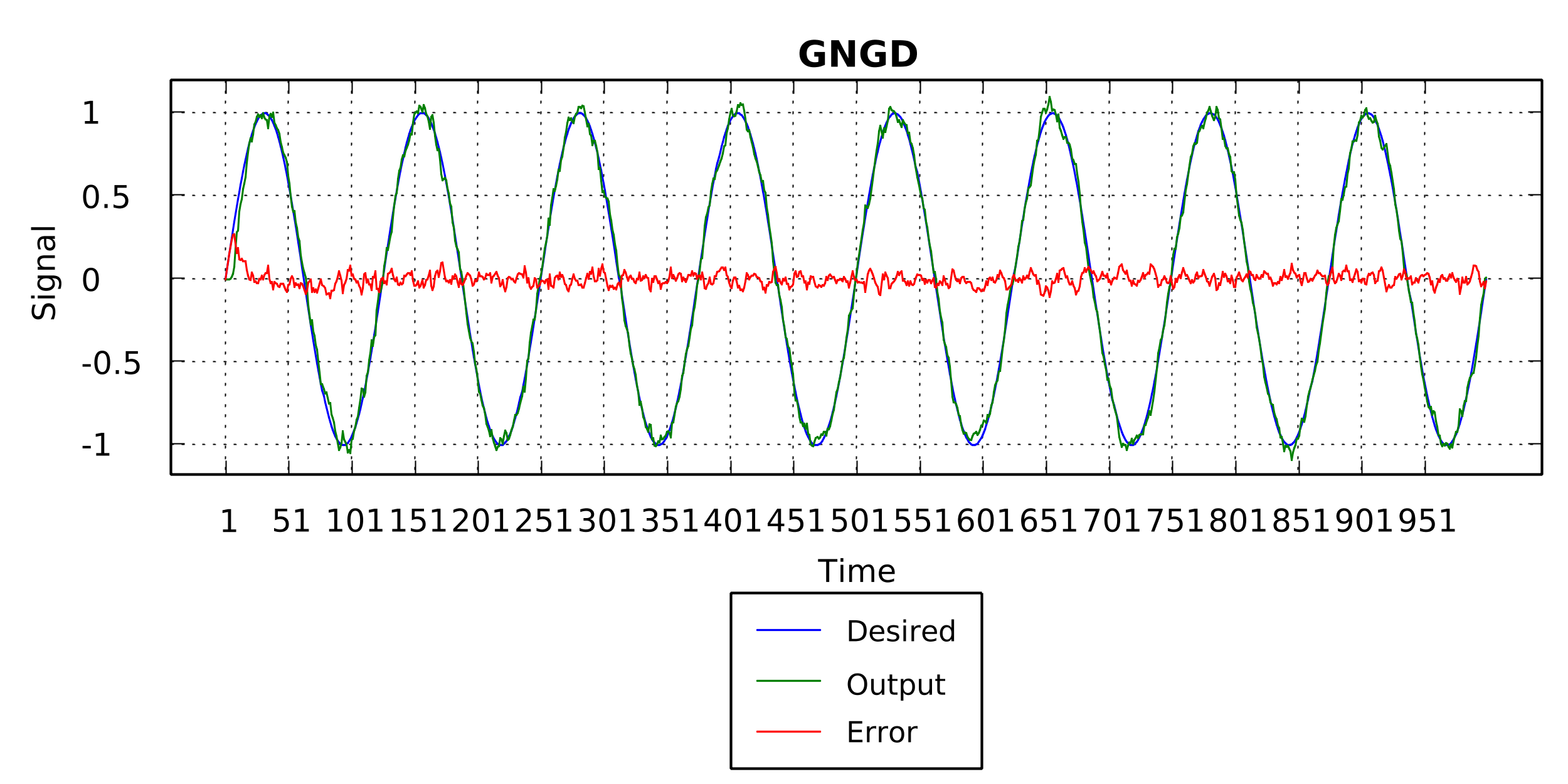```                    ```
int order = 3;
double mu = 0.25;
double eps = 0.001;
int length = 20;

GNGD filt1 = new GNGD(length, mu, eps, ro, GNGD.WeightsFillMethod.ZEROS); // Initialising weights to zero
filt1.filter(desired, signal);
```
```

## Least Mean Squares Adaptive Filter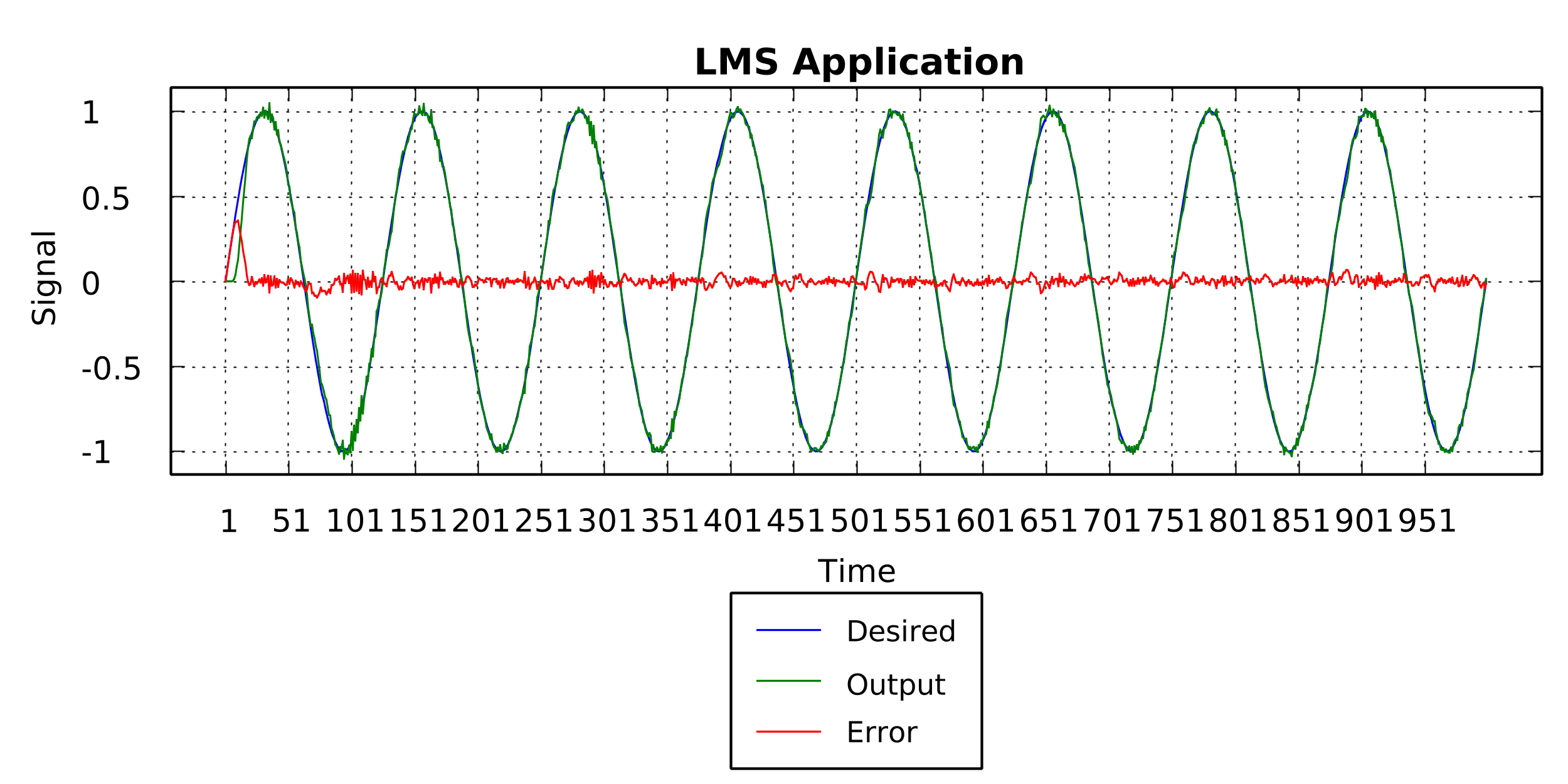```                    ```
double mu = 0.1;
int length = 20;

LMS filt1 = new LMS(mu, length, LMS.WeightsFillMethod.ZEROS); // Initialising weights to zero
filt1.filter(desired, signal);
```
```

## Normalised Least Mean Squares Adaptive Filter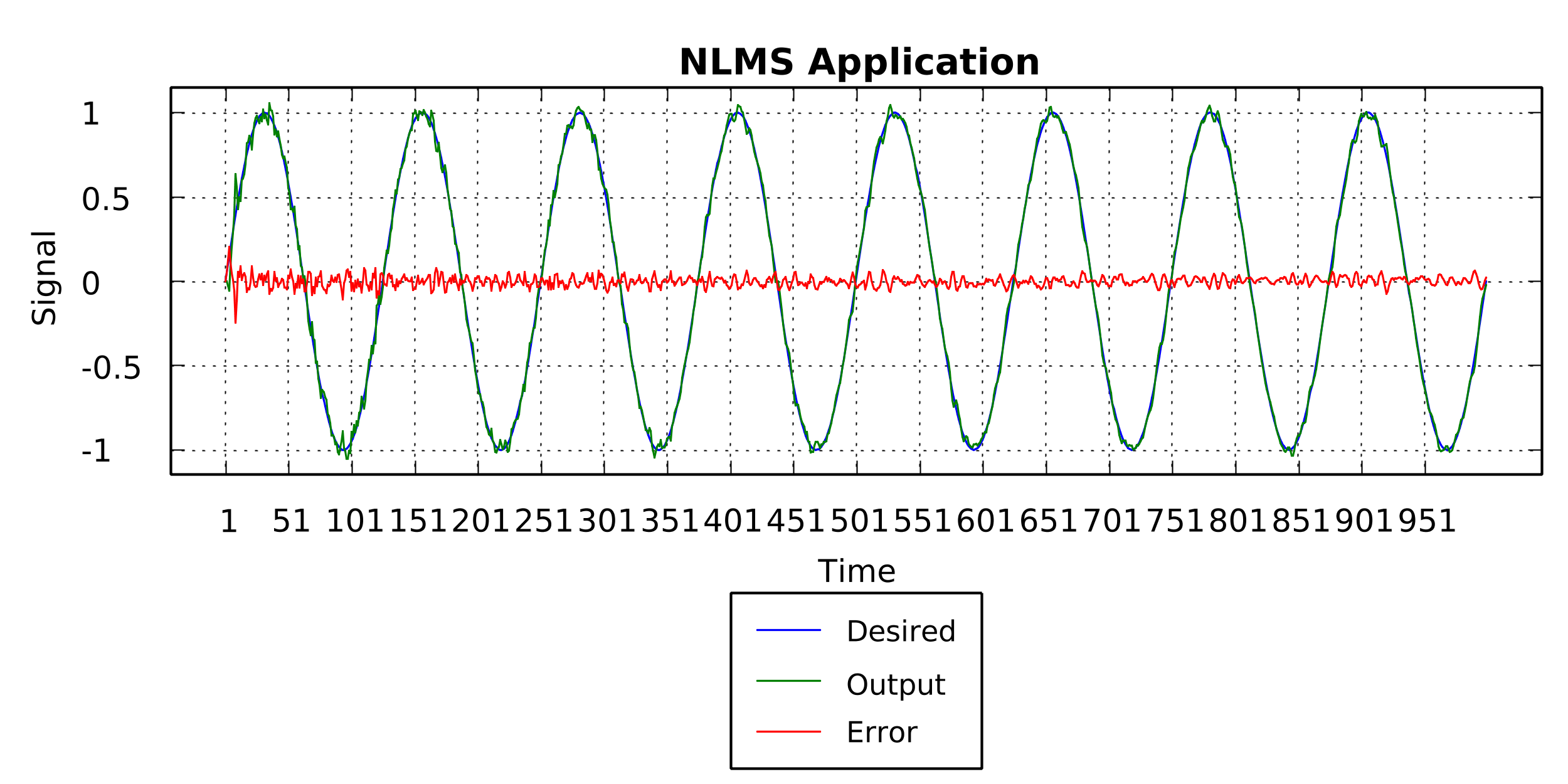```                    ```
double mu = 1.0;
int length = 20;

NLMS filt1 = new NLMS(mu, length, NLMS.WeightsFillMethod.ZEROS); // Initialising weights to zero
filt1.filter(desired, signal);
```
```

## Reduced Least Squares Adaptive Filter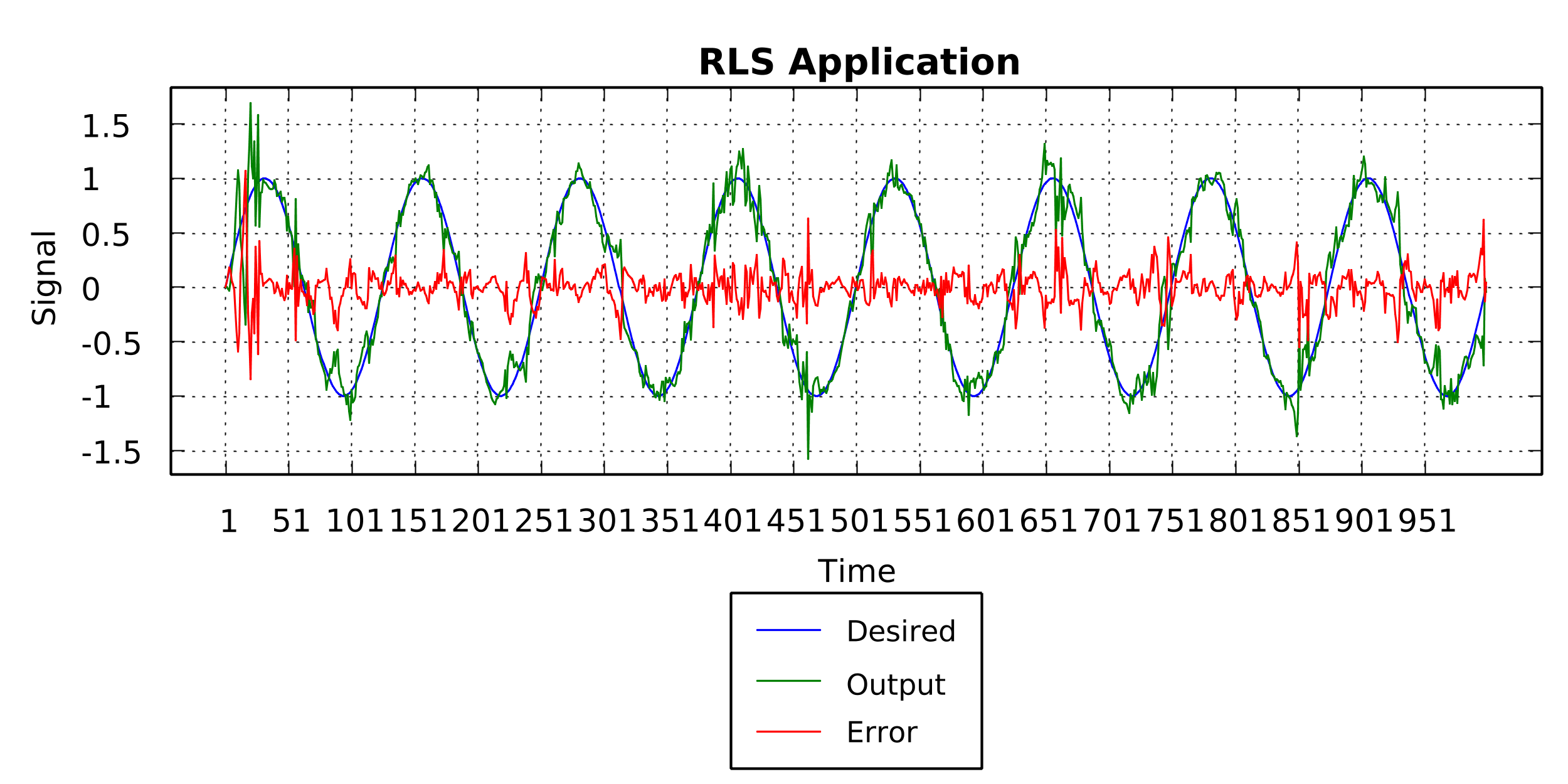```                    ```
double mu = 0.5;
double eps = 0.25;
int length = 20;

RLS filt1 = new RLS(length, mu, eps, RLS.WeightsFillMethod.ZEROS); // Initialising weights to zero
filt1.filter(desired, signal);
```
```

## Sign-Sign Least Mean Squares Adaptive Filter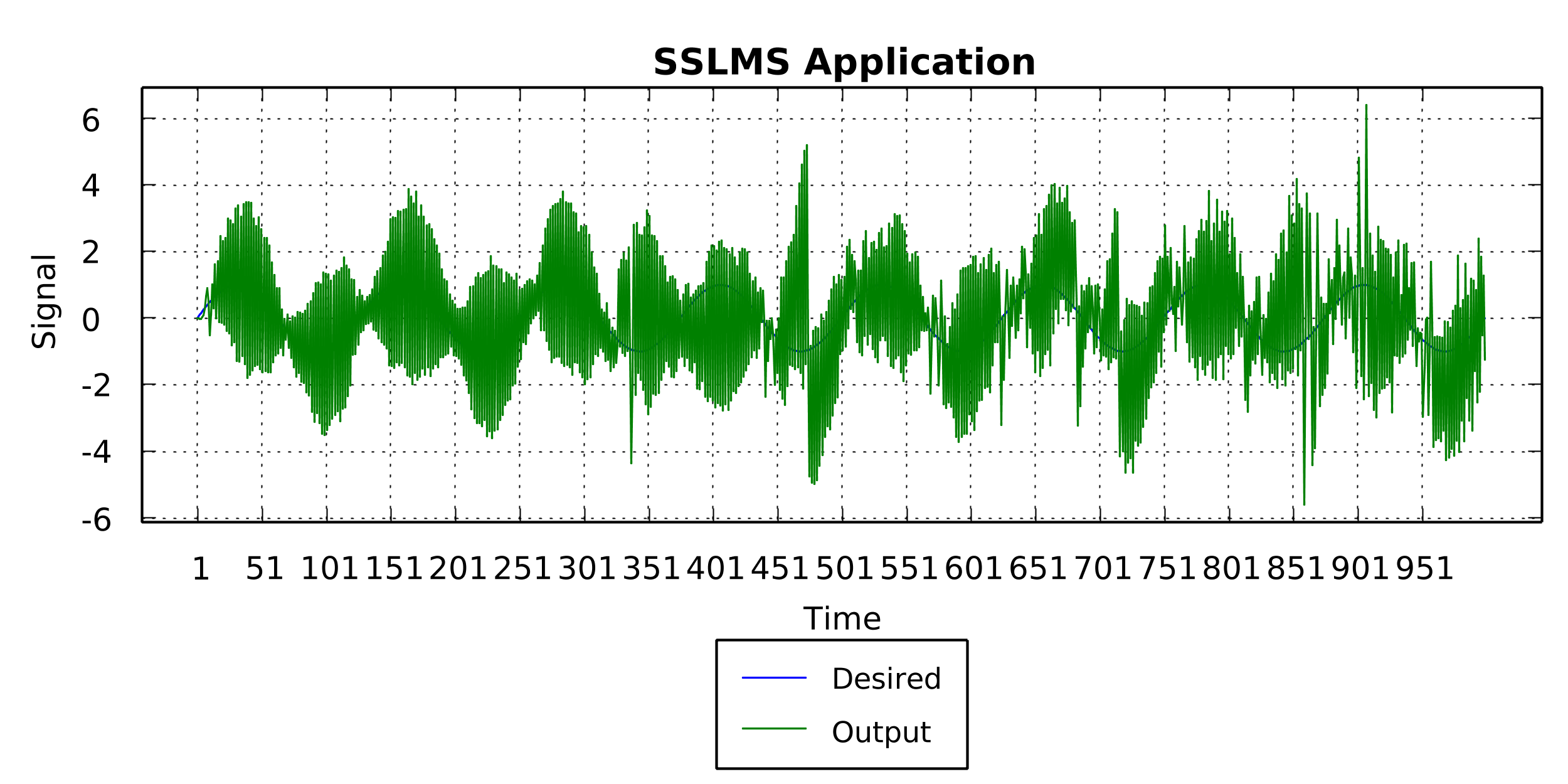```                    ```
double mu = 0.25;
int length = 20;

SSLMS filt1 = new SSLMS(mu, length, SSLMS.WeightsFillMethod.ZEROS); // Initialising weights to zero
filt1.filter(desired, signal);
```
```

## Normalised Sign-Sign Least Mean Squares Adaptive Filter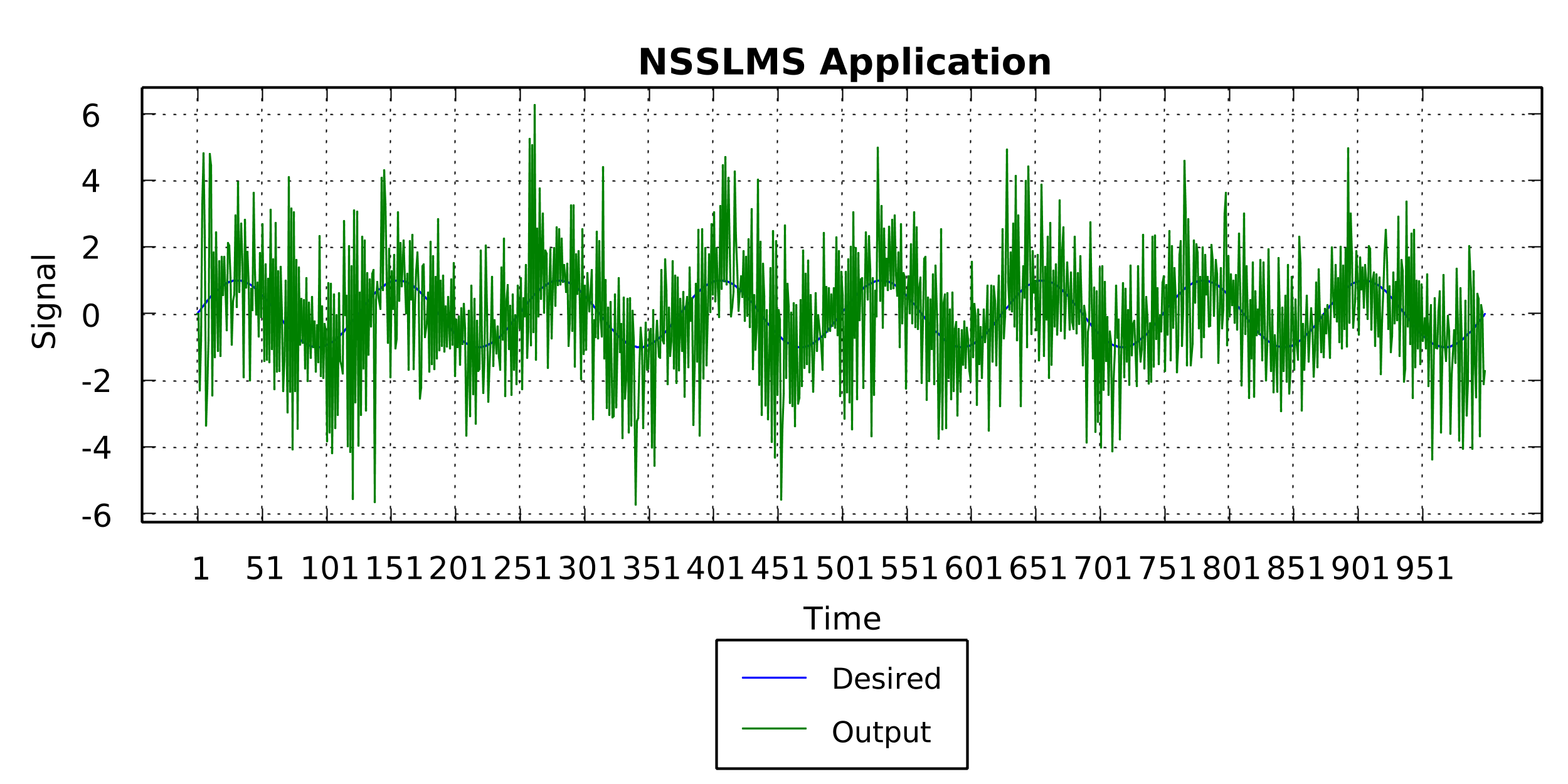```                    ```
double mu = 1.0;
int length = 20;

NSSLMS filt1 = new NSSLMS(mu, length, SSLMS.WeightsFillMethod.ZEROS); // Initialising weights to zero
filt1.filter(desired, signal);
```
```